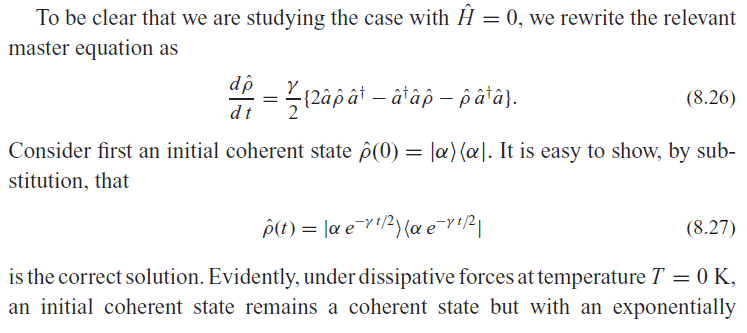# How to solve this master equation?

• Tspirit

## Homework Statement

In Gerry's book "Introductory quantum optics", Chapter 8.5 Decoherence, Page 207, there is a calculation which doesn't give the detail derivation, who can give me the calculation from (8.26) to (8.27)?## Homework Equations

The coherent state is defined by $$\hat{a}|\alpha>=\alpha|\alpha>$$.

## The Attempt at a Solution

Traditionally, we can calculate the differential equation of a complex function. However, it is about an equation of operator and the order of the operators is very important. I have no idea about it.

#### Attachments

They are saying that if you substitute their formula for ##\rho## into the left side of their equation equation for ##\frac{d\rho}{dt}## then it equals the right-hand side. Of course ##\frac{d\rho}{dt}=\frac{d|\alpha e^{-\frac{\gamma t}{2}}>}{dt}<\alpha e^{-\frac{\gamma t}{2}}|+
|\alpha e^{\frac{-\gamma t}{2}}>\frac
{d <\alpha e^
{-\frac{\gamma t}{2}}|
}
{dt}##
Can you provide a bit of background? What is the time dependence of ##|\alpha>##?